# Aligned Induction

## Induced modelling of digit

MNIST - handwritten digits/Induced modelling of digit

### Sections

Introduction

15-fud model

All pixels

Averaged pixels

Array of square regions of 15x15 pixels

Two level over 10x10 regions

Two level over 15x15 regions

Two level over centred square regions of 11x11 pixels

### Introduction

We have considered using just the substrate variables to predict digit, $V_{\mathrm{k}} \to V_{\mathrm{l}}$, by minimising the label entropy or query conditional entropy. See Entropy and alignment. We found that, overall, of all of these models the 511-mono-variate-fud has the highest accuracy of 75.5%.

We have gone on to consider various ways of creating an unsupervised induced model $D$ on the query variables, $V_{\mathrm{k}}$, which exclude digit. Now we shall analyse this model, $D$, to find a semi-supervised submodel that predicts the label variables, $V_{\mathrm{l}}$, or digit. That is, we shall search in the decomposition fud for a submodel that optimises conditional entropy.

There are some examples of model induction in the NISTPy repository.

### 15-fud model

We have created a 15-fud induced model, $D$, over all pixels NIST_model2.json of 7,500 events of the training sample, see Model 2 and Model 2.

from NISTDev import *

(uu,hrtr) = nistTrainBucketedIO(2)

digit = VarStr("digit")
vv = uvars(uu)
vvl = sset([digit])
vvk = vv - vvl

hr = hrev([i for i in range(hrsize(hrtr)) if i % 8 == 0],hrtr)

df = dfIO('./NIST_model2.json')

uu1 = uunion(uu,fsys(dfff(df)))

len(dfund(df))
134

len(fvars(dfff(df)))
515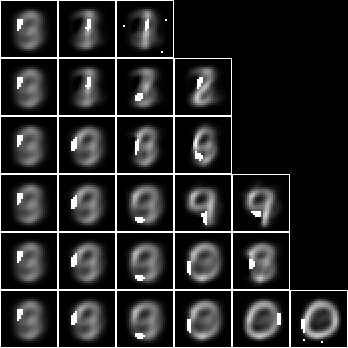This induced model is not interested particularly in digit, but the leaf nodes tend to resemble particular digits nonetheless. Consider this model as a predictor of label. Create the fud decomposition fud, $F = D^{\mathrm{F}}$,

ff = dfnul(uu1,df,1)

uu2 = uunion(uu,fsys(ff))

hrb = hrfmul(uu2,ff,hr)



The label entropy, $\mathrm{lent}(A * \mathrm{his}(F^{\mathrm{T}}),W_F,V_{\mathrm{l}})$, where $W_F = \mathrm{der}(F)$, is

hrlent(uu2,hrb,fder(ff),vvl)
1.4174827406154242


Load the test sample and select a subset of 1000 events $A_{\mathrm{te}}$,

(_,hrte) = nistTestBucketedIO(2)

hrq = hrev([i for i in range(hrsize(hrte)) if i % 10 == 0],hrte)

hrqb = hrfmul(uu2,ff,hrq)



The conditional entropy decomposition fud of a bi-valent substrate is mostly effective, $\mathrm{size}((A_{\mathrm{te}} * F^{\mathrm{T}}) * (A * F^{\mathrm{T}})^{\mathrm{F}}) \approx \mathrm{size}(A_{\mathrm{te}})$,

size(mul(hhaa(hrhh(uu2,hrhrred(hrqb,fder(ff)))),eff(hhaa(hrhh(uu2,hrhrred(hrb,fder(ff)))))))
# 988 % 1


Overall, this model is correct for 43.6% of the test sample, $\begin{eqnarray} &&|\{R : (S,\cdot) \in A_{\mathrm{te}} * \mathrm{his}(F^{\mathrm{T}})~\%~(W_F \cup V_{\mathrm{l}}),~Q = \{S\}^{\mathrm{U}}, \\ &&\hspace{4em}R = A * \mathrm{his}(F^{\mathrm{T}})~\%~(W_F \cup V_{\mathrm{l}}) * (Q\%W_F),~\mathrm{size}(\mathrm{max}(R) * (Q\%V_{\mathrm{l}})) > 0\}| \end{eqnarray}$

def amax(aa):
ll = aall(norm(trim(aa)))
ll.sort(key = lambda x: x, reverse = True)
return llaa(ll[:1])

len([rr for (_,ss) in hhll(hrhh(uu2,hrhrred(hrqb,fder(ff)|vvl))) for qq in [single(ss,1)] for rr in [araa(uu2,hrred(hrhrsel(hrb,hhhr(uu2,aahh(red(qq,fder(ff))))),vvl))] if size(rr) > 0 and size(mul(amax(rr),red(qq,vvl))) > 0])
436


So the accuracy is similar to the 5-tuple (44.8%) and considerably lower than the 1-tuple 15-fud (56.7%).

We can test the accuracy of model 2 in a compiled executable, see NIST test, with these results,

model: NIST_model2
train size: 7500
model cardinality: 515
nullable fud cardinality: 688
nullable fud derived cardinality: 147
nullable fud underlying cardinality: 134
ff label ent: 1.4174827406154242
test size: 1000
effective size: 988
matches: 436


The underlying of the 15-mono-variate-fud conditional entropy model is just the query substrate, $V_{\mathrm{k}}$. Now let us consider taking the fud decomposition fud variables, $\mathrm{vars}(F)$, of the induced model, $F = D^{\mathrm{F}}$, as the underlying. First we must reframe the fud variables $F_1$,

def refr1():
def refr1_f(v):
if isinstance(v, VarPair):
(w,i) = v._rep
if isinstance(w, VarPair):
(f,l) = w._rep
return VarPair((VarPair((VarPair((VarInt(1),f)),l)),i))
return v
return refr1_f

def tframe(f,tt):
reframe = transformsMapVarsFrame
nn = sdict([(v,f(v)) for v in tvars(tt)])
return reframe(tt,nn)

def fframe(f,ff):
return qqff([tframe(f,tt) for tt in ffqq(ff)])

ff1 = fframe(refr1(),ff)

uu1 = uunion(uu,fsys(ff1))



Now we apply the reframed fud to the sample, $A_1 = A * \mathrm{his}(F_1^{\mathrm{T}})$,

hr1 = hrfmul(uu1,ff1,hr)



Now apply the conditional entropy fud decomper to minimise the label entropy. We will construct a 15-fud conditional model over the 15-fud induced model, $\{D_2\} = \mathrm{leaves}(\mathrm{tree}(Z_{P,A_1,\mathrm{L,D,F}}))$,

def decompercondrr(ll,uu,aa,kmax,omax,fmax):

(kmax,omax) = (1,5)

(uu2,df2) = decompercondrr(vvl,uu1,hr1,kmax,omax,15)

dfund(df2)
# {<16,16>, <17,16>, <<<1,3>,1>,18>, <<<1,3>,1>,21>, <<<1,3>,1>,47>, <<<1,4>,n>,1>, <<<1,4>,1>,6>, <<<1,6>,1>,35>, <<<1,7>,n>,4>, <<<1,12>,1>,16>, <<<1,12>,2>,33>, <<<1,12>,2>,35>, <<<1,14>,1>,35>}

len(dfund(df2))
13

def dfll(df):
return treesPaths(dfzz(df))

rpln([[fund(ff) for (_,ff) in ll] for ll in dfll(df2)])
# [{<<<1,4>,1>,6>}, {<<<1,3>,1>,21>}, {<<<1,12>,2>,33>}, {<<<1,6>,1>,35>}]
# [{<<<1,4>,1>,6>}, {<<<1,3>,1>,21>}, {<<<1,12>,1>,16>}]
# [{<<<1,4>,1>,6>}, {<<<1,3>,1>,21>}, {<17,16>}, {<16,16>}]
# [{<<<1,4>,1>,6>}, {<<<1,3>,1>,21>}, {<17,16>}, {<<<1,12>,2>,35>}]
# [{<<<1,4>,1>,6>}, {<<<1,12>,1>,16>}, {<<<1,3>,1>,18>}]
# [{<<<1,4>,1>,6>}, {<<<1,12>,1>,16>}, {<<<1,14>,1>,35>}]
# [{<<<1,4>,1>,6>}, {<<<1,7>,n>,4>}, {<<<1,12>,1>,16>}, {<<<1,3>,1>,47>}]
# [{<<<1,4>,1>,6>}, {<<<1,4>,n>,1>}]


We can see that the conditional entropy fud decomper has chosen fud variables rather than substrate variables, with two exceptions, <17,16> and <16,16>.

Let us get the underlying model dependencies $D’_2$,

df21 = zzdf(funcsTreesMap(lambda xx:(xx,fdep(xx|ff1,fder(xx))),dfzz(df2)))



Imaging the slices of the decomposition $P = \mathrm{paths}(A * D’_2)$,

pp = treesPaths(hrmult(uu2,df21,hr))

def fid(ff):
return variablesVariableFud(fder(ff))

rpln([[fid(ff) for ((_,ff),_) in ll] for ll in pp])
# [1, 2, 5, 11]
# [1, 2, 13]
# [1, 2, 6, 14]
# [1, 2, 6, 12]
# [1, 3, 7]
# [1, 3, 9]
# [1, 4, 8, 15]
# [1, 10]

rpln([[hrsize(hr) for (_,hr) in ll] for ll in pp])
# [7500, 3077, 1363, 786]
# [7500, 3077, 467]
# [7500, 3077, 1247, 715]
# [7500, 3077, 1247, 532]
# [7500, 2092, 877]
# [7500, 2092, 713]
# [7500, 1445, 748, 414]
# [7500, 886]

file = "NIST.bmp"

bmwrite(file,ppbm2(uu,vvk,28,2,2,pp))

bmwrite(file,ppbm(uu,vvk,28,2,2,pp))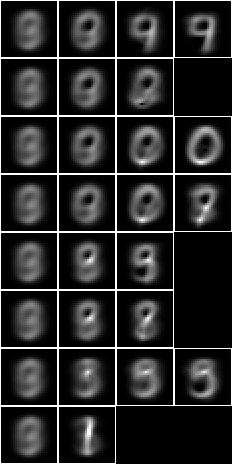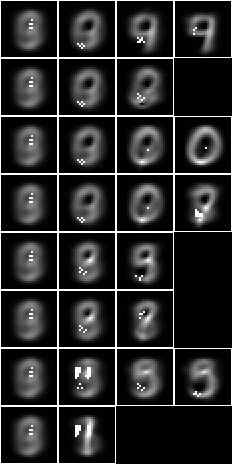Let us apply the 2-level 15-fud to the test sample to calculate the accuracy of prediction. First we will construct the dependent fud decomposition fud $F_2 = D_2^{‘\mathrm{F}}$,

ff2 = dfnul(uu2,df21,2)

len(fvars(ff2))
200

uu2 = uunion(uu1,fsys(ff2))

len(uvars(uu2))
1393


The underlying, $\mathrm{und}(F_2)$, is shown overlaid on the average, $\hat{A}\%V_{\mathrm{k}}$,

hrbmav = hrbm(28,3,2,hrhrred(hr,vvk))

bmwrite(file,bmborder(1,bmmax(hrbmav,0,0,hrbm(28,3,2,qqhr(2,uu,vvk,fund(ff2))))))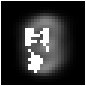We can see that the 2-level 15-fud covers more of the substrate than the 15-mono-variate-fud.

Consider this model, $D’_2$, as a predictor of digit,

ww2 = fder(ff2)

hr2 = hrfmul(uu2,ff2,hr)



The label entropy, $\mathrm{lent}(A * \mathrm{his}(F_2^{\mathrm{T}}),W_2,V_{\mathrm{l}})$, where $W_2 = \mathrm{der}(F_2)$, is

hrlent(uu2,hr2,ww2,vvl)
1.3719639739083007


This model is effective for all of the test sample, $\mathrm{size}((A_{\mathrm{te}} * F_2^{\mathrm{T}}) * (A * F_2^{\mathrm{T}})^{\mathrm{F}}) \approx \mathrm{size}(A_{\mathrm{te}})$,

hrq2 = hrfmul(uu2,ff2,hrq)

size(mul(hhaa(hrhh(uu2,hrhrred(hrq2,ww2))),eff(hhaa(hrhh(uu2,hrhrred(hr2,ww2))))))
# 1000 % 1


It is correct for 46.0% of events, $\begin{eqnarray} &&|\{R : (S,\cdot) \in A_{\mathrm{te}} * \mathrm{his}(F_2^{\mathrm{T}})~\%~(W_2 \cup V_{\mathrm{l}}),~Q = \{S\}^{\mathrm{U}}, \\ &&\hspace{4em}R = A * \mathrm{his}(F_2^{\mathrm{T}})~\%~(W_2 \cup V_{\mathrm{l}}) * (Q\%W_2),~\mathrm{size}(\mathrm{max}(R) * (Q\%V_{\mathrm{l}})) > 0\}| \end{eqnarray}$

def amax(aa):
ll = aall(norm(trim(aa)))
ll.sort(key = lambda x: x, reverse = True)
return llaa(ll[:1])

len([rr for (_,ss) in hhll(hrhh(uu2,hrhrred(hrq2,ww2|vvl))) for qq in [single(ss,1)] for rr in [araa(uu2,hrred(hrhrsel(hr2,hhhr(uu2,aahh(red(qq,ww2)))),vvl))] if size(rr) > 0 and size(mul(amax(rr),red(qq,vvl))) > 0])
460


This is less accurate than the 1-tuple 15-fud (56.7%), but higher than just model 2 by itself (43.6%). The clusters of the 2-level conditional over induced model tend to be a bit more spread out than the 1-level induced model. Note that the pure 1-level conditional model only has a single pixel per fud, rather than clusters.

We can run the conditional entropy fud decomper in an engine. Given NIST_model2.json, the engine creates NIST_model36.json, see Model 36,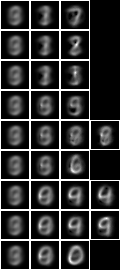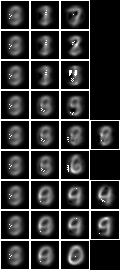We can then test the accuracy of model 36 in a compiled executable, see NIST test, with these results,

model: NIST_model36
train size: 7500
model cardinality: 162
nullable fud cardinality: 202
nullable fud derived cardinality: 15
nullable fud underlying cardinality: 63
ff label ent: 1.4004486362258195
test size: 1000
effective size: 1000
matches: 507


Note that the statistics differ slightly from those calculated above, because the engine uses the entire training dataset of 60,000 events.

### All pixels

Now let us repeat the analysis of Model 2, but for the 127-fud induced model of all pixels, see Model 35.

First let us test the accuracy of the induced model by itself in NIST test,

model: NIST_model35
train size: 7500
model cardinality: 4040
nullable fud cardinality: 5597
nullable fud derived cardinality: 1316
nullable fud underlying cardinality: 488
ff label ent: 0.8203986651817567
test size: 1000
effective size: 946
matches: 612


This model is correct for 61.2% of the test sample, which may be compared to Model 2 (50.7%). When compared to the non-modelled case it is more accurate than the 15-mono-variate-fud (56.7%), but less accurate than the 127-mono-variate-fud (70.0%).

Again, we can run the conditional entropy fud decomper to create a 2-level 127-fud, NIST_model37.json, see Model 37,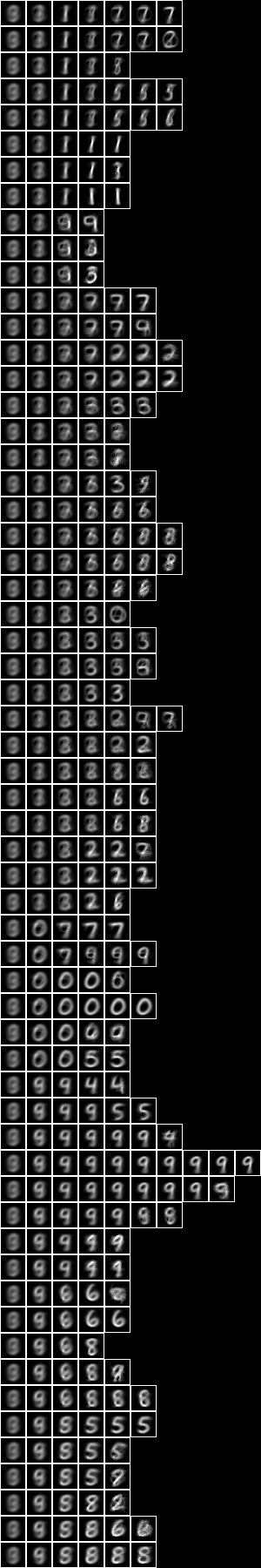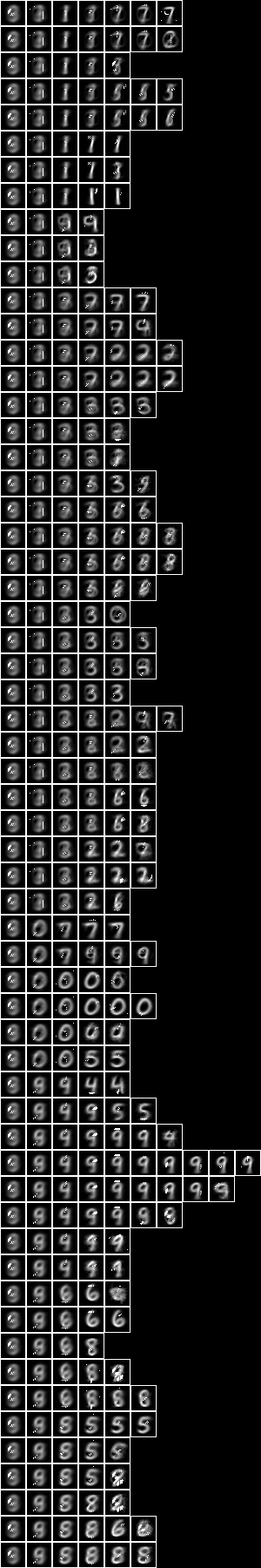Testing the accuracy of model 37,

model: NIST_model37
train size: 7500
model cardinality: 663
nullable fud cardinality: 1039
nullable fud derived cardinality: 127
nullable fud underlying cardinality: 253
ff label ent: 0.6564998305260028
test size: 1000
effective size: 999
matches: 773


So the accuracy of 77.3% is higher than the 127-bi-variate-fud (74.0%) and the 511-mono-variate-fud (75.5%). Here we have demonstrated that a semi-supervised induced model can be more predictive of label (77.3%) than either the unsupervised induced model, $D$, (61.2%) or the non-induced model (74.0%).

Again, the clusters of the conditional over induced model look a bit more spread out than the 1-level induced model.

Repeating the same, but to create a 2-tuple conditional over 127-fud, NIST_model39.json, see Model 39,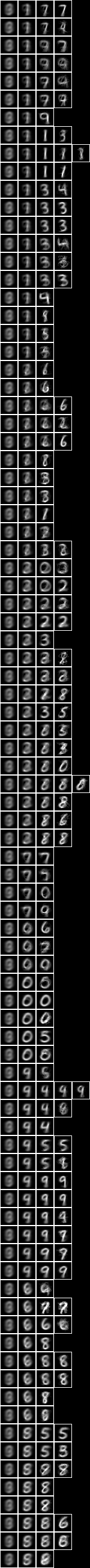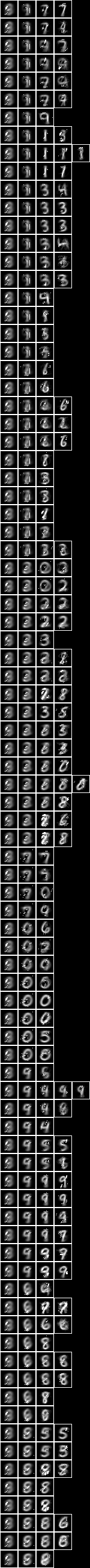Testing the accuracy of model 39,

model: NIST_model39
train size: 7500
model cardinality: 872
nullable fud cardinality: 1242
nullable fud derived cardinality: 127
nullable fud underlying cardinality: 301
ff label ent: 0.4036616947586324
test size: 1000
effective size: 979
matches: 797


The accuracy of the bi-variate has increased to 79.7%. It is not as great an increase as we saw between the 127-mono-variate-fud (70.0%) and the 127-bi-variate-fud (74.0%).

The clusters of the bi-variate conditional over induced model look still more spread out than the 1-level induced model.

The accuracy of the semi-supervised sub-model can be increased by obtaining larger samples, for example by random affine variation, and then inceasing the depth of the decomposition to 511-fud or 1023-fud.

### Averaged pixels

Now let us repeat the conditional entropy analysis for the 127-fud induced model of averaged pixels, see Model 34.

First let us test the accuracy of model 34 in NIST test averaged,

model: NIST_model34
train size: 7500
model cardinality: 4553
nullable fud cardinality: 6089
nullable fud derived cardinality: 1294
nullable fud underlying cardinality: 70
ff label ent: 2.2037648474789409
test size: 1000
effective size: 1000
matches: 156


This model is correct for only 15.6% of the test sample, which is much less than Model 35 (61.2%).

Again, we can run the conditional entropy fud decomper to create a 2-level 127-fud, NIST_model38.json, see Model 38,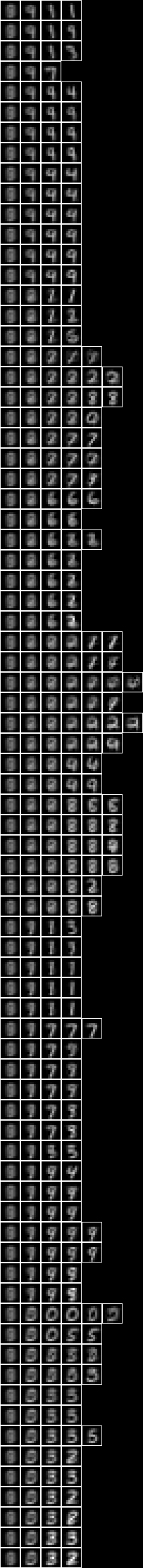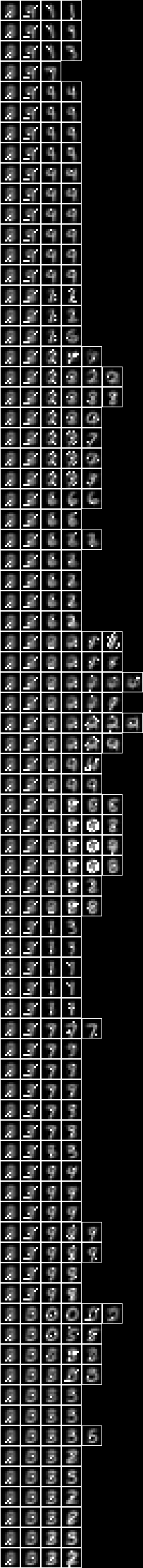Testing the accuracy of model 38,

model: NIST_model38
selected train size: 7500
model cardinality: 308
nullable fud cardinality: 683
nullable fud derived cardinality: 127
nullable fud underlying cardinality: 45
ff label ent: 0.6309812112866986
test size: 1000
effective size: 986
matches: 718


So the accuracy of the semi-supervised induced model, 71.8%, is higher than that of the unsupervised induced model, $D$, (15.6%), but not as high as the non-averaged all pixels model (77.3%).

### Array of square regions of 15x15 pixels

Now let us repeat the conditional entropy analysis of all pixels, but for a model consisting of an array of 7x7 127-fud induced models of square regions of 15x15 pixels, see Model 21.

When we run the regional conditional entropy fud decomper to create a conditional model, NIST_model43.json, see Model 43,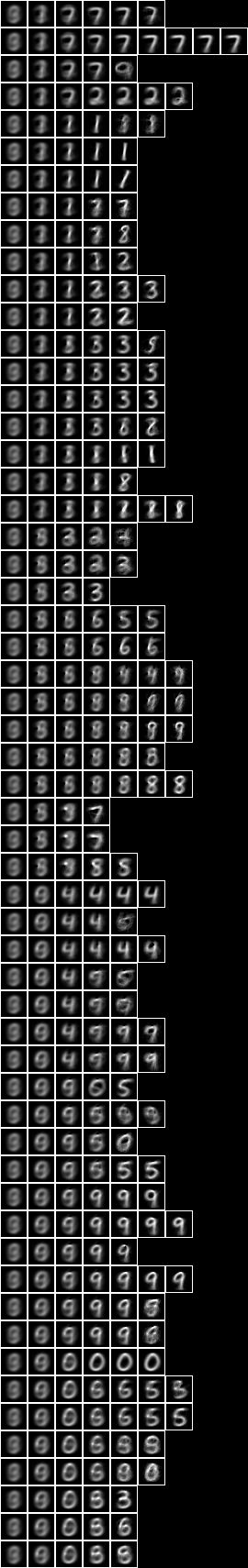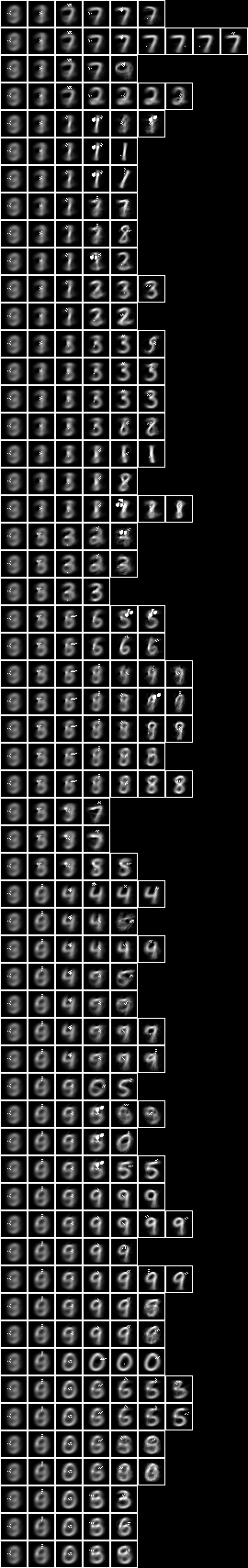Testing the accuracy of model 43,

model: NIST_model43
train size: 7500
model cardinality: 868
nullable fud cardinality: 1244
nullable fud derived cardinality: 127
nullable fud underlying cardinality: 218
ff label ent: 0.6758031753055889
test size: 1000
effective size: 999
matches: 770


The accuracy of 77.0% is similar to the all pixels (77.3%) above.

### Two level over 10x10 regions

Now let us repeat the conditional entropy analysis of all pixels, but for the 2-level model over square regions of 10x10 pixels, see Model 24.

When we run the conditional entropy fud decomper to create a conditional model, NIST_model40.json, see Model 40,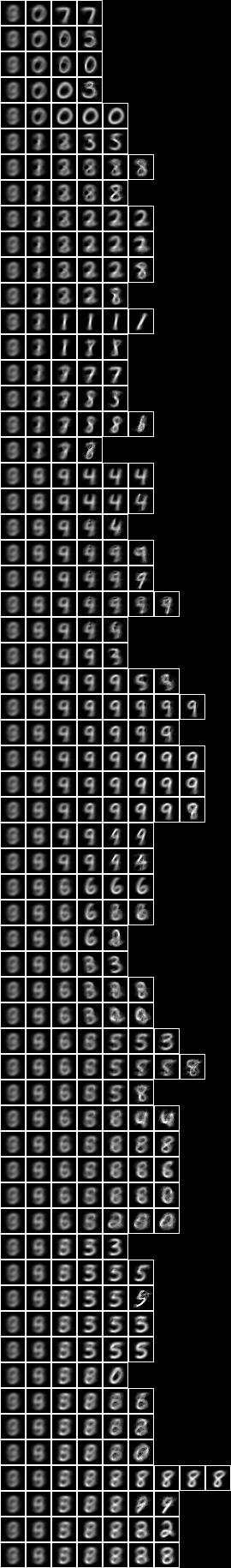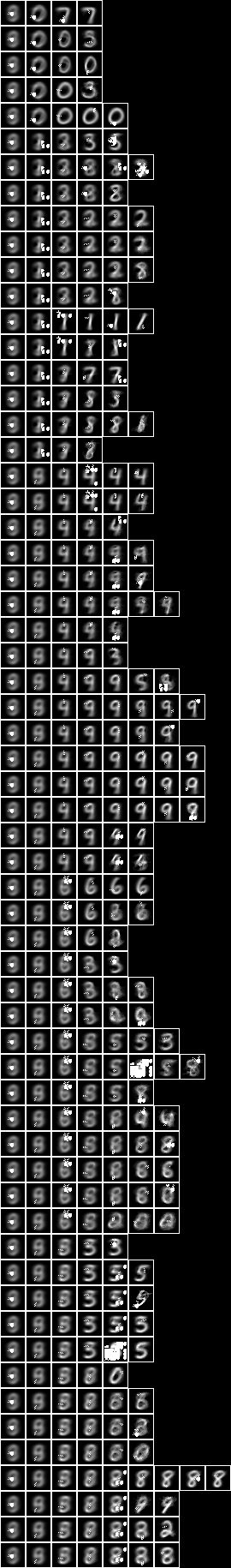Testing the accuracy of model 40,

model: NIST_model40
train size: 7500
model cardinality: 2140
nullable fud cardinality: 2516
nullable fud derived cardinality: 127
nullable fud underlying cardinality: 562
ff label ent: 0.5836689910897102
test size: 1000
effective size: 997
matches: 802


The accuracy of 80.2% is similar to the all pixels (77.3%) above.

### Two level over 15x15 regions

Now let us repeat the conditional entropy analysis of all pixels, but for the 2-level model over square regions of 15x15 pixels, see Model 25.

When we run the conditional entropy fud decomper to create a conditional model, NIST_model41.json, see Model 41,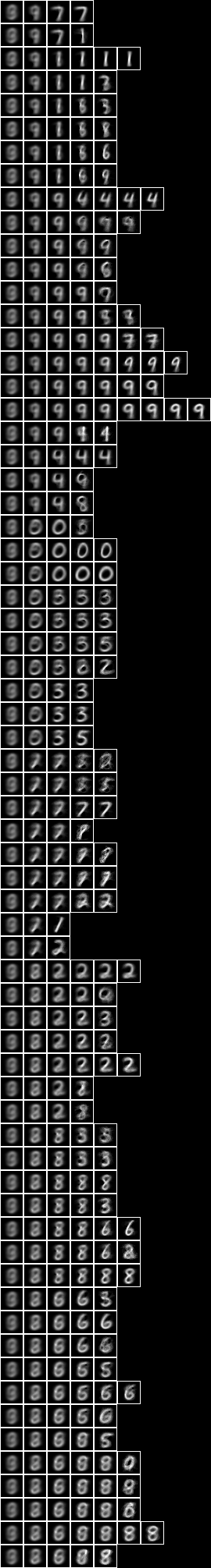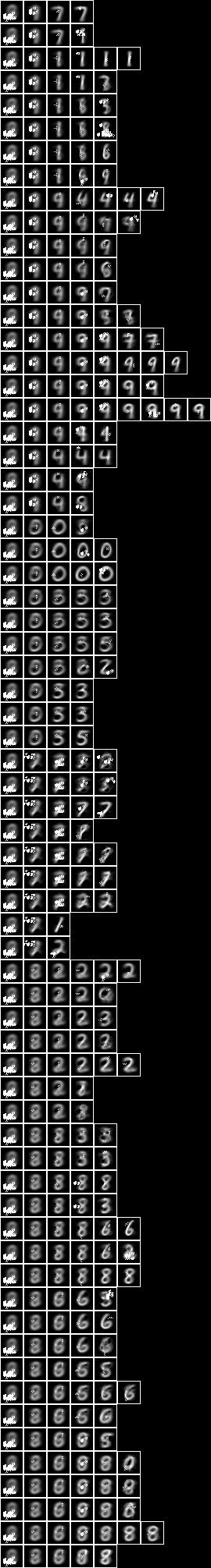Testing the accuracy of model 41,

model: NIST_model41
train size: 7500
model cardinality: 1264
nullable fud cardinality: 1639
nullable fud derived cardinality: 127
nullable fud underlying cardinality: 421
ff label ent: 0.5874380803591093
test size: 1000
effective size: 998
matches: 778


The accuracy of 77.8% is similar to the all pixels (77.3%) and the two level over 10x10 regions (80.2%) above.

### Two level over centred square regions of 11x11 pixels

Now let us repeat the conditional entropy analysis of all pixels, but for the 2-level model over centred square regions of 11x11 pixels, see Model 26.

When we run the conditional entropy fud decomper to create a conditional model, NIST_model42.json, see Model 42,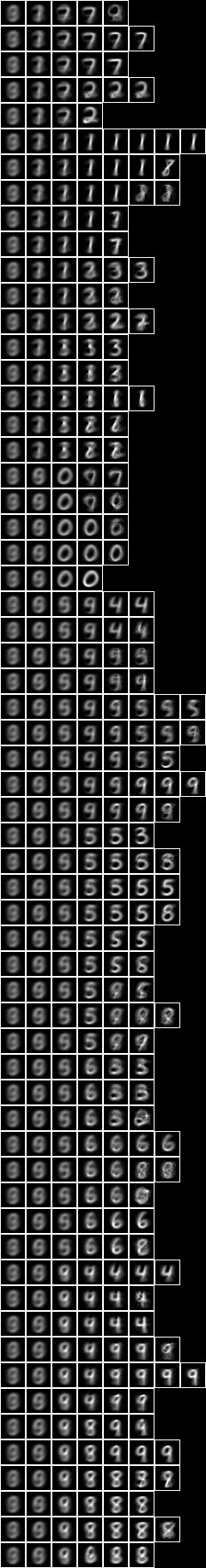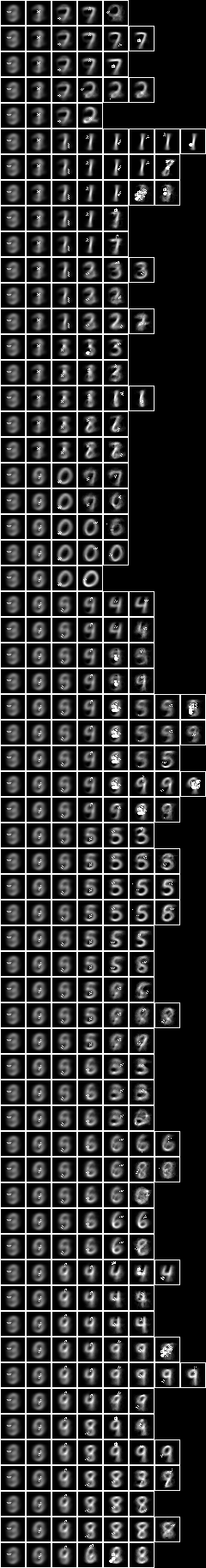Testing the accuracy of model 42,

model: NIST_model42
train size: 7500
model cardinality: 772
nullable fud cardinality: 1149
nullable fud derived cardinality: 127
nullable fud underlying cardinality: 274
ff label ent: 0.6004072422056215
test size: 1000
effective size: 1000
matches: 785


The accuracy of 78.5% is similar to the all pixels (77.3%), the two level over 10x10 regions (80.2%), and the two level over 15x15 regions (77.8%) above.

In general, although the 2-level induced models that are based on underlying regional levels have higher alignments than the 1-level induced model, these extra alignments are not particularly related to digit, and so the higher level features are not very prominent in the resulting semi-supervised sub-models.

top